CBSE Class 12 Sample Paper for 2022 Boards (MCQ Based - for Term 1)

Class 12
Solutions of Sample Papers and Past Year Papers - for Class 12 Boards

## (a) x/√(1 - x 2 )    (b) 1/√(1 - x 2 ) (c) 1/√(1 + x 2 )   (d) x/√(1 + x 2 )

This question is inspired from Misc 15 (MCQ) - Chapter 2 Class 12 - Inverse Trigonometric Functions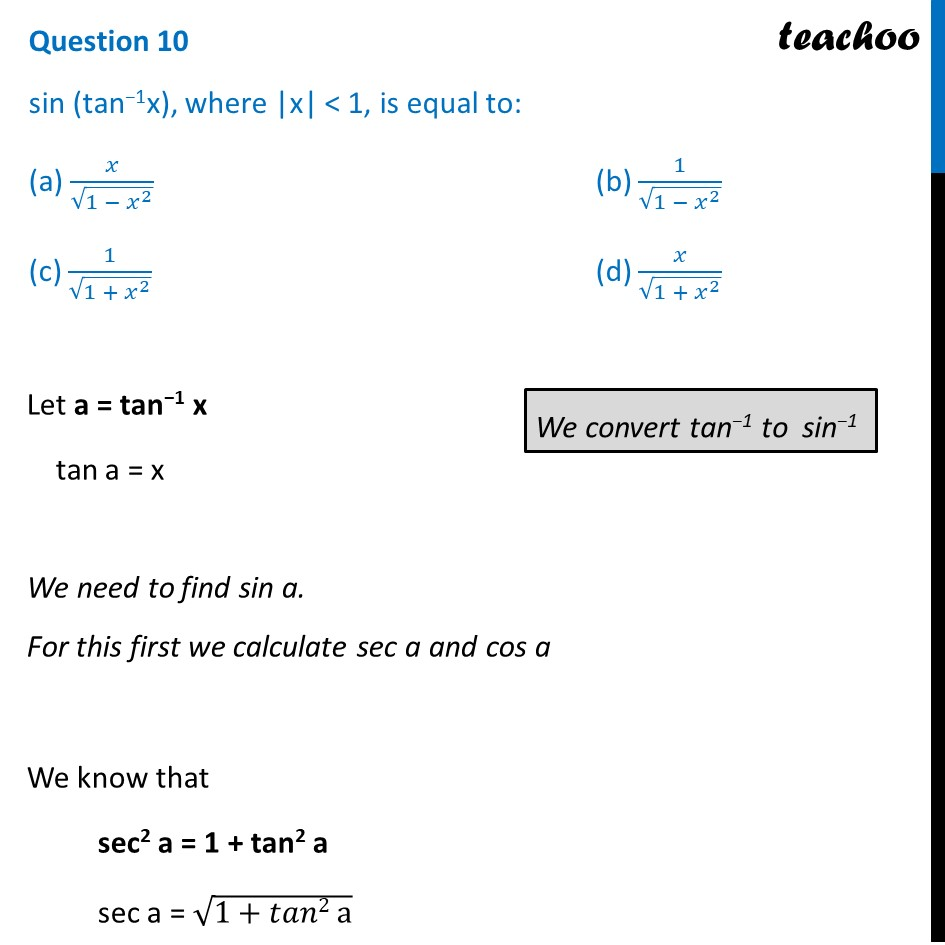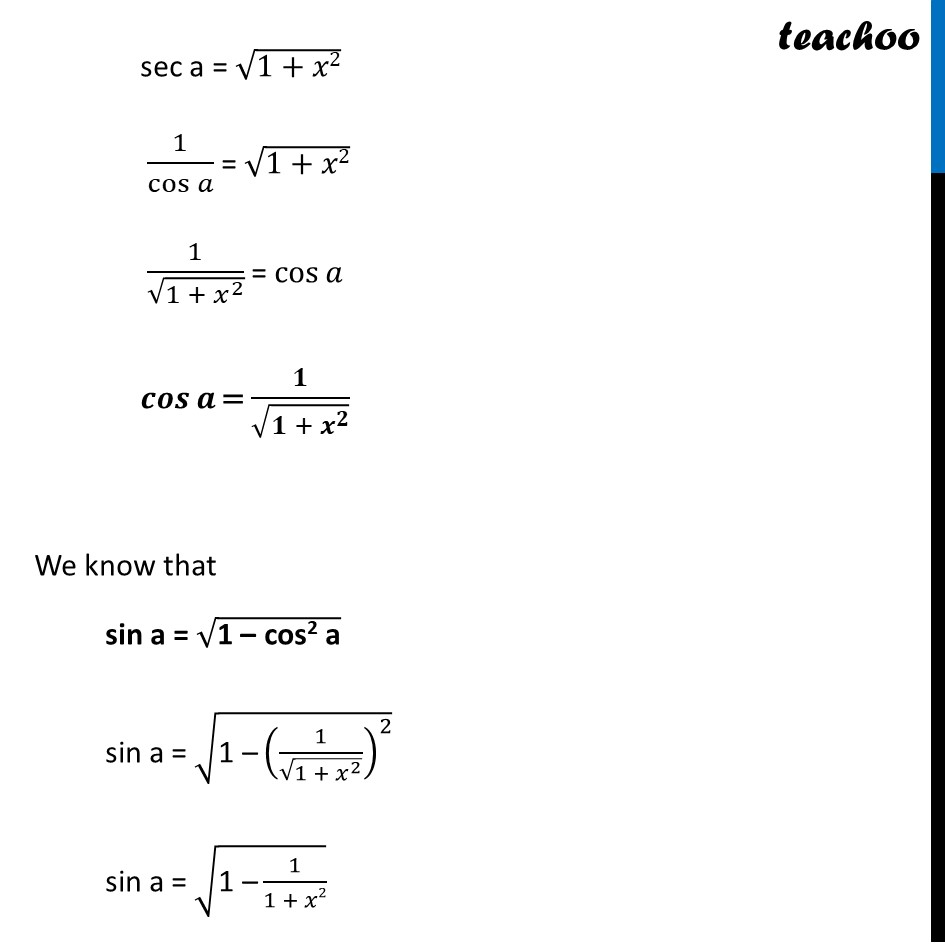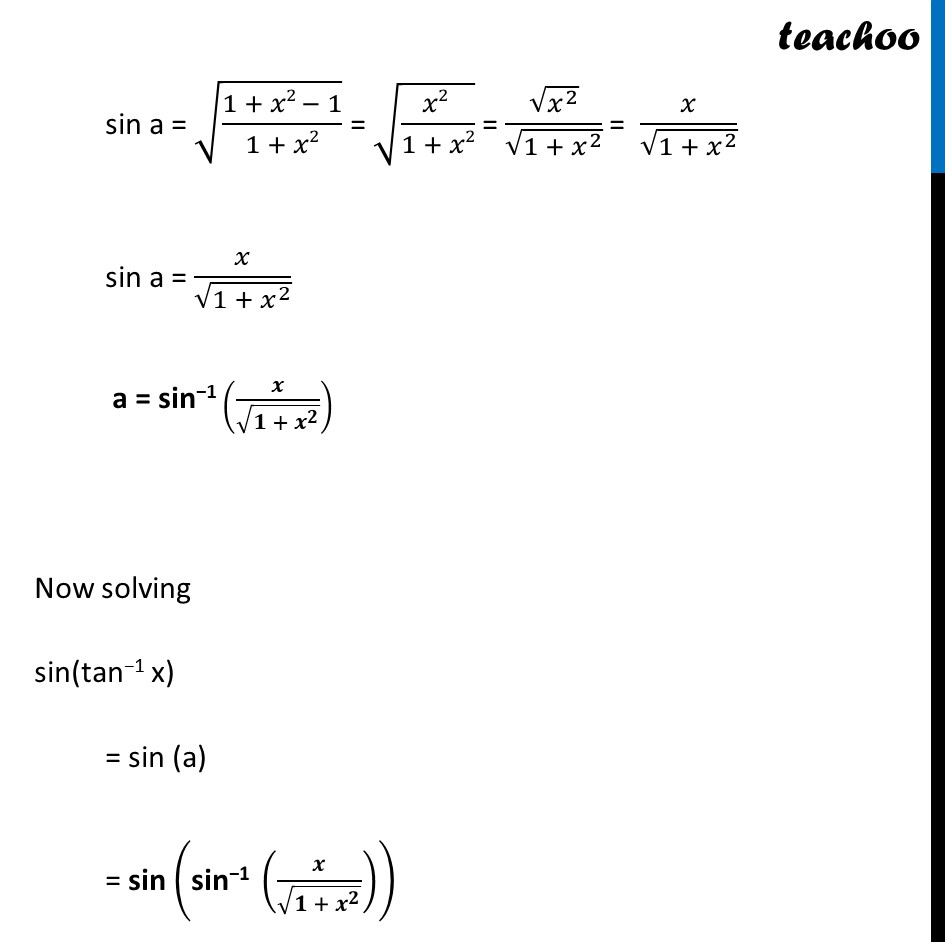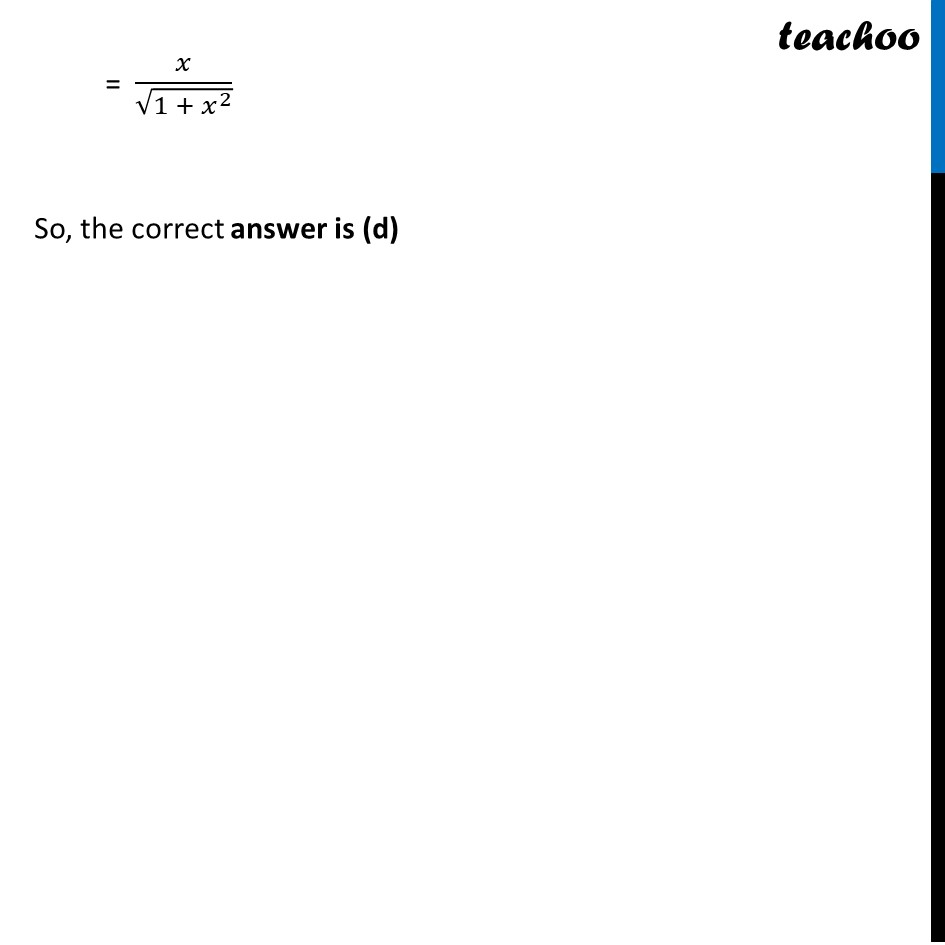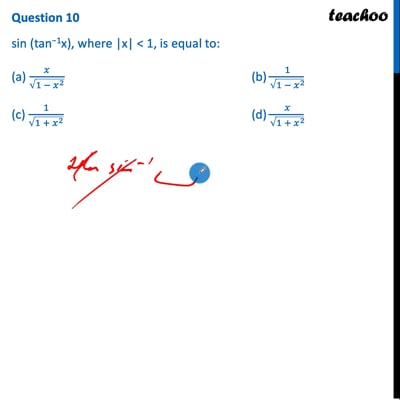This video is only available for Teachoo black users

Introducing your new favourite teacher - Teachoo Black, at only ₹83 per month

### Transcript

Question 10 sin (tan−1x), where |x| < 1, is equal to: (a) 𝑥/√(1 − 𝑥^2 ) (b) 1/√(1 −〖 𝑥〗^2 ) (c) 1/√(1 + 𝑥^2 ) (d) 𝑥/√(1 + 𝑥^2 ) Let a = tan−1 x tan a = x We need to find sin a. For this first we calculate sec a and cos a We know that sec2 a = 1 + tan2 a sec a = √(1+𝑡𝑎𝑛2 a) sec a = √(1+𝑥2) 1/cos⁡𝑎 = √(1+𝑥2) 1/√(1 + 𝑥^2 ) = cos⁡𝑎 𝒄𝒐𝒔⁡𝒂 = 𝟏/√(𝟏 + 𝒙^𝟐 ) We know that sin a = √("1 – cos2 a" ) sin a = √("1 –" (1/√(1 + 𝑥^2 ))^2 ) sin a = √("1 –" 1/(1 + 𝑥2)) sin a = √((1 + 𝑥2 − 1)/(1 + 𝑥2)) = √((𝑥2 )/(1 + 𝑥2)) = √(𝑥^2 )/√(〖1 + 𝑥〗^2 ) = 𝑥/√(〖1 + 𝑥〗^2 ) sin a = 𝑥/√(〖1 + 𝑥〗^2 ) a = sin−1 (𝒙/√(〖𝟏 + 𝒙〗^𝟐 )) Now solving sin(tan−1 x) = sin (a) = sin ("sin−1 " (𝒙/√(〖𝟏 + 𝒙〗^𝟐 ))) = 𝑥/√(〖1 + 𝑥〗^2 ) So, the correct answer is (d)